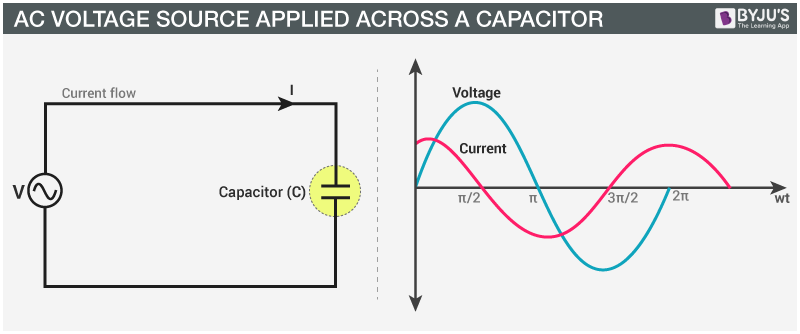# AC Voltage Source Applied Across a Capacitor

We know that a capacitor is a passive electronic device with two terminals. It has the capacity to store electrical energy in an electrical field. In a DC circuit, when a capacitor is connected to a voltage source, current will flow for the short time required to charge the capacitor. In this section, we will learn the expression of the AC voltage source applied across a capacitor in detail.

## AC Voltage Source Applied Across a Capacitor

Let us consider the electric circuit shown below. We have a capacitor and an AC voltage V, represented by the symbol ~, that produces a potential difference across its terminals that varies sinusoidally. Here, the potential difference or the AC voltage can be given as,

$$\begin{array}{l}v=v_{m}\; sin\; \omega t\end{array}$$

Here, vm is the amplitude of the oscillating potential difference and the angular frequency is given by ω. The current through the resistor due to the present voltage source can be calculated using the Kirchhoff’s loop rule, as under,

$$\begin{array}{l}\sum V(t)=0\end{array}$$For the given capacitor we can write,

$$\begin{array}{l}v=\frac{q}{C}\end{array}$$

According to Kirchhoff’s rule, we can write from the above circuit,

$$\begin{array}{l}v_{m}\; sin\; \omega t=\frac{q}{C}\end{array}$$

The current through the circuit can be calculated using the relation,

$$\begin{array}{l}i=\frac{dq}{dt}\\ \\ \Rightarrow i=\frac{d(v_{m}\; Csin\; \omega t)}{dt}=\omega Cv_{m}\; cos\; \omega t\\ \\ \Rightarrow i=i_{m}sin(\omega t+\frac{\pi }{2})\; \; [Using\; the \; relation,\; cos\; \omega t=sin(\omega t+\frac{\pi }{2}) ]\end{array}$$

Here the amplitude of the current can be written as,

$$\begin{array}{l}i_{m}=\omega Cv_{m}\end{array}$$

or else we can write it as,

$$\begin{array}{l}i_{m}=\frac{v_{m}}{\frac{1}{\omega _{C}}}\end{array}$$

Here, we can see that the term 1/ωC can be said to be equivalent to the resistance of this device and is termed as the capacitive resistance. We denote the capacitive resistance of the device as XC.

$$\begin{array}{l}X_{C}=\frac{1}{\omega _{C}}\end{array}$$

And thus, we can say that the amplitude of the current in this circuit is given as,

$$\begin{array}{l}i_{m}=\frac{v_{m}}{X_{C}}\end{array}$$

In the above equations, the dimension of the capacitive resistance can be seen to be the same as that of resistance, and also, the SI unit of capacitance is given as ohm. The capacitive resistance restricts the passage of current in a purely capacitive circuit the same way as resistance hinders the passage of current in a purely resistive circuit.

Here we say, that the capacitive resistance is inversely proportional to the frequency and the capacitance. We also see from the above equations that the current in a capacitive circuit is π/2 ahead of the voltage across the capacitor.

The instantaneous power supplied to the capacitor can be given in terms of the current passing through the capacitor as,

$$\begin{array}{l}P_{c}=iv=i_{m}\; cos\; \omega t\: v_{m}\; sin\; \omega t\\ \\ P_{c}=\frac{i_{m}v_{m}}{2}sin2\omega t\end{array}$$

Here, the average power supplied over a complete cycle can be given as,

$$\begin{array}{l}P=\frac{i_{m}v_{m}}{2}sin2\omega t=0\end{array}$$

Concluding the article, we can say that in the case of a capacitor the current leads the voltage by π/2.
Read more: AC voltage applied to an inductor

## Frequently Asked Questions – FAQs

### What is AC current?

An electric current that reverses direction periodically and changes its magnitude continuously with respect to time is known as Alternating current (AC).

### What is the SI unit of capacitance?

The SI unit of capacitance is Ohm.

### State Kirchhoff’s Voltage Law.

Kirchhoff’s Voltage Law states that “according to the loop rule, the sum of the voltages around the closed loop is equal to null.”

True.

### What is the capacitive resistance of the device XC?

XC =1/ωC

Stay tuned with BYJU’S to explore interesting topics on the electric circuit, AC Voltage, and many more. Join us and fall in love with learning.